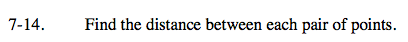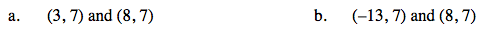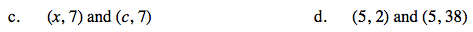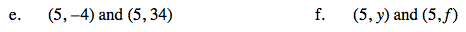### Home > AC > Chapter 7 > Lesson 7.1.2 > Problem7-14

7-14.
1. Find the distance between each pair of points. Homework Help ✎

1. (3, 7) and (8, 7)

2. (−13, 7) and (8, 7)

3. (x, 7) and (c, 7)

4. (5, 2) and (5, 38)

5. (5, −4) and (5, 34)

6. (5, y) and (5, f)Graph the points.

Use the same method as in part (a).

21 units awayHow do you find the distance between two numbers?

I xc I units away

Graph the points. How is this different from part (a)?Use the same method as in part (d).

Use the same methods as in part (c).